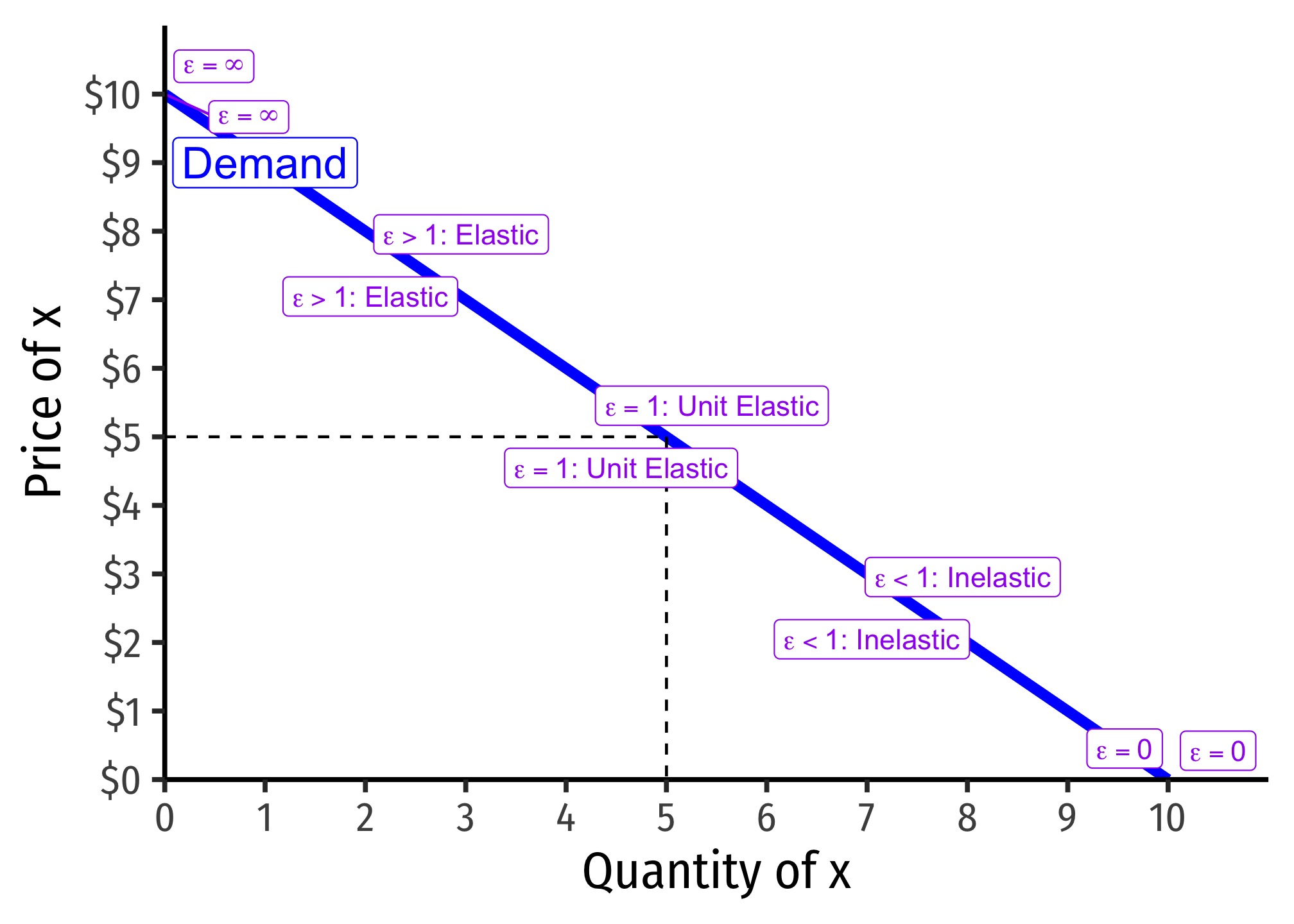Meeting Date

Tuesday, February 7, 2023

## Overview

We begin looking at monopoly by discussing how a monopolist (as compared to a price-taking firm) chooses both its output and its price to maximize profits.

## Practice

Today you will be working on practice problems:

## Slides

Below, you can find the slides in two formats. Clicking the image will bring you to the html version of the slides in a new tab. The lower button will allow you to download a PDF version of the slides.

Tip

You can type h to see a special list of viewing options, and type o for an outline view of all the slides.

I suggest printing the slides beforehand and using them to take additional notes in class (not everything is in the slides)!

## Appendix

### Price Elasticity of Demand Refresher

Price elasticity of demand measures how much (in %) quantity demanded changes in response to a (1%) change in price.

\begin{align*} \epsilon_{q,p} &= \frac{\% \Delta q}{\% \Delta p}\\ &= \cfrac{\left(\frac{\Delta q}{q}\right)}{\left(\frac{\Delta p}{p}\right)}\\ &= \frac{\Delta q}{\Delta p} \times \frac{p}{q}\\ &= \frac{1}{slope} \times \frac{p}{q}\\ \end{align*}

“Elastic” “Unit Elastic” “Inelastic”
Intuitively: Large response Proportionate response Little response
Mathematically: $$\vert \epsilon_{q_D,p}\vert > 1$$ $$\vert \epsilon_{q_D,p}\vert = 1$$ $$\vert \epsilon_{q_D,p} \vert < 1$$
Numerator $$>$$ Denominator Numerator $$=$$ Denominator Numerator $$<$$ Denominator
A 1% change in $$p$$ More than 1% change in $$q_D$$ 1% change in $$q_D$$ Less than 1% change in $$q_D$$

Price elasticity of demand changes along the demand curve:#### Determinants of Price Elasticity

What determines how responsive your buying behavior is to a price change?

• More (fewer) substitutes $$\implies$$ more (less) elastic
• Larger categories of products (less elastic) vs. specific brand (more elastic)
• Necessities (less elastic) vs. luxuries (more elastic)
• Large (more elastic) vs. small (less elastic) portion of budget
• More (less) time to adjust $$\implies$$ more (less) elastic

### Derivation of the Lerner Index

Marginal revenue is strongly related to the price elasticity of demand, which is $$E_{D}=\frac{\Delta q}{\Delta p} \times \frac{p}{q}$$1

We derived marginal revenue (in the slides) as: $MR(q)=p+\frac{\Delta p}{\Delta q}q$

Firms will always maximize profits where:

\begin{align*} MR(q)&=MC(q) && \text{Profit-max output}\\ p+\bigg(\frac{\Delta p}{\Delta q}\bigg)q&=MC(q) && \text{Definition of } MR(q)\\ p+\bigg(\frac{\Delta p}{\Delta q}\bigg) q \times \frac{p}{p}&=\frac{MR(q)}{p} && \text{Multiplying the left by } \frac{p}{p} \text{ (i.e. 1)}\\ p+\underbrace{\bigg(\frac{\Delta p}{\Delta q}\times \frac{q}{p} \bigg)}_{\frac{1}{\epsilon}} \times p &=MC(q) && \text{Rearranging the left}\\ p+\frac{1}{\epsilon} \times p &=MC(q) && \text{Recognize price elasticity } \epsilon=\frac{\Delta q}{\Delta p} \times \frac{p}{q}\\ p &=MC(q) - \frac{1}{\epsilon} p && \text{Subtract }\frac{1}{\epsilon}p \text{ from both sides}\\ p-MC(q) &= -\frac{1}{\epsilon} p && \text{Subtract }MC(q) \text{ from both sides}\\ \frac{p-MC(q)}{p} &= -\frac{1}{\epsilon} && \text{Divide both sides by }p\\ \end{align*}

The left side gives us the fraction of price that is markup $$\left(\frac{p-MC(q)}{p}\right)$$, and the right side shows this is inversely related to price elasticity of demand.

## Footnotes

1. I sometimes simplify it as $$E_{D}=\frac{1}{slope} \times \frac{p}{q}$$, where “slope” is the slope of the inverse demand curve (graph), since the slope is $$\frac{\Delta p}{\Delta q} = \frac{rise}{run}$$.↩︎